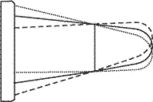# mean chord

## mean chord

[′mēn ′kȯrd]
(aerospace engineering)
That chord of an airfoil that is equal to the sum of all the airfoil's chord lengths divided by the number of chord lengths; equivalently, that chord whose length is equal to the area of the airfoil section divided by the span.
McGraw-Hill Dictionary of Scientific & Technical Terms, 6E, Copyright © 2003 by The McGraw-Hill Companies, Inc.

## mean chordThe mean geometric chord location is the same for two extreme positions of the root chord.
i. The chord of an imaginary wing of a constant section having the same force vectors under all conditions as those of the actual wing. That chord of an airfoil is equal to the sum of all the airfoil's chord lengths divided by the number of chord lengths. Equivalently, it is that chord whose length is equal to the area of the airfoil section divided by the span.
ii. Sometimes wrongly referred to as a mean aerodynamic chord.
References in periodicals archive ?
where [bar.C] is the mean chord length of a convex region of area A bounded by a curve L of length L, and [bar.w] is the mean width of L - recall Eq.
As an example, let us mention the formula for the mean chord length [bar.C] of a convex region of volume V, bounded by a closed convex surface S of surface area S:
The rated wind speed is 9m/sec, the wind wheel diameter is 2.3 m, the rated rotational speed is 350 r / min and the mean chord length is 20cm.
Payn expresses his concern that the new fighter's high centre of gravity in relation to the equivalent mean chord meant it might not be manoeuvrable enough.
When fibres flocculate or fillers deposit on fibres, the mean chord length increases.
After flocculant addition, the mean chord length changes with time and the maximum value (maximum mean chord size), increases from about 12 [micro]m to over 40 [micro]m with PEO dosage.
Figure 4 shows the effect on the mean chord size when cPAM is added.
The cPAM-induced pulp flocculation is strongly affected by salt, as can be seen from Figure 10; by increasing the salt concentration (NaCl) from zero to 5 g/L (60 mM), the maximum mean chord size is much reduced as expected for the cationic retention aids.
Up to a dosage of 0.1 mg of PEI/g, salt has little effect on the mean chord size and even when the plateau is eventually reached the mean chord size remains small.
Then the mean chord length determined in [B.sub.R] by the corresponding p-line is,

Site: Follow: Share:
Open / Close